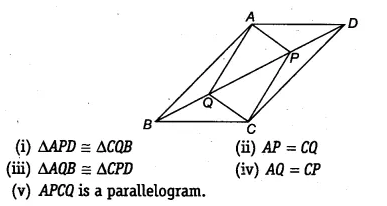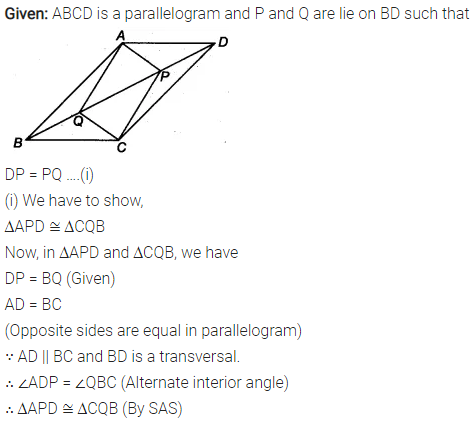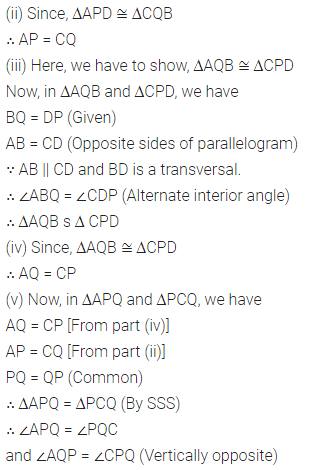# In parallelogram ABCD, two points P and Q are taken on diagonal BD such that DP = BQ

In parallelogram ABCD, two points P and Q are taken on diagonal BD such that DP = BQ (see figure). Show that